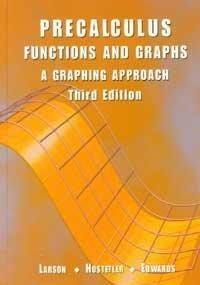174984

9780618052905

# Pre Calculus Functions and Graphs: A Graphing Approach\$12.55
\$3.95 Shipping
List Price
\$125.56
Discount
90% Off
You Save
\$113.01

• Condition: Acceptable
• Provider: Read A Book Contact
• Provider Rating:
85%
• Ships From: Multiple Locations
• Shipping: Standard
• Comments: IMP: Acceptable- Do not include ACCESS CODE, CD-ROM or companion materials even if stated in item title. It may contain highlighting/markings throughout, and the covers and corners may show shelf wear. Corners, pages may be dent. All text is legible. B30-day money back guarantee

Most renters respond to questions in 48 hours or less.
The response will be emailed to you.
• ISBN-13: 9780618052905
• ISBN: 0618052909
• Edition: 3rd
• Publisher: Houghton Mifflin College Div

### AUTHOR

Bruce H. Edwards, Ron Larson, Robert P. Hostetler

### SUMMARY

Contents Note: Each chapter includes a Chapter Summary, Review Exercises, and a Chapter Test. P. Prerequisites P.1 Graphical Representation of Data P.2 Graphs of Equations P.3 Lines in the Plane P.4 Solving Equations Algebraically and Graphically P.5 Solving Inequalities Algebraically and Graphically Chapter Project: Modeling the Volume of a Box Library of Functions 1. Functions and Their Graphs 1.1 Functions 1.2 Graphs of Functions 1.3 Shifting, Reflecting, and Stretching Graphs 1.4 Combinations of Functions 1.5 Inverse Functions Chapter Project: Modeling the Area of a Plot 2. Polynomial and Rational Functions 2.1 Quadratic Functions 2.2 Polynomial Functions of Higher Degree 2.3 Real Zeros of Polynomial Functions 2.4 Complex Numbers 2.5 The Fundamental Theorem of Algebra 2.6 Rational Functions and Asymptotes 2.7 Graphs of Rational Functions Chapter Project: Finding Points of Intersection 3. Exponential and Logarithmic Functions 3.1 Exponential Functions and Their Graphs 3.2 Logarithmic Functions and Their Graphs 3.3 Properties of Logarithms 3.4 Solving Exponential and Logarithmic Equations 3.5 Exponential and Logarithmic Models Chapter Project: A Graphical Approach to Compound Interest Cumulative Test: Chapters 1-3 4. Trigonometric Functions 4.1 Radian and Degree Measure 4.2 Trigonometric Functions: The Unit Circle 4.3 Right Triangle Trigonometry 4.4 Trigonometric Functions of Any Angle 4.5 Graphs of Sine and Cosine Functions 4.6 Graphs of Other Trigonometric Functions 4.7 Inverse Trigonometric Functions 4.8 Applications and Models Chapter Project: Fitting a Model to Data 5. Analytic Trigonometry 5.1 Using Fundamental Identities 5.2 Verifying Trigonometric Identities 5.3 Solving Trigonometric Equations 5.4 Sum and Difference Formulas 5.5 Multiple-Angle and Product-Sum Formulas Chapter Project: Projectile Motion 6. Additional Topics in Trigonometry 6.1 Law of Sines 6.2 Law of Cosines 6.3 Vectors in the Plane 6.4 Vectors and Dot Products 6.5 Trigonometric Form of a Complex Number Chapter Project: Adding Vectors Graphically Cumulative Test: Chapters 4-6 7. Systems of Equations and Inequalities 7.1 Solving Systems of Equations 7.2 Systems of Linear Equations in Two Variables 7.3 Multivariable Linear Systems 7.4 Systems of Inequalities 7.5 Linear Programming Chapter Project: Fitting Models to Data 8. Matrices and Determinants 8.1 Matrices and Systems of Equations 8.2 Operations with Matrices 8.3 The Inverse of a Square Matrix 8.4 The Determinant of a Square Matrix 8.5 Applications of Matrices and Determinants Chapter Project: Solving Systems of Equations 9. Sequences, Series, and Probability 9.1 Sequences and Series 9.2 Arithmetic Sequences and Partial Sums 9.3 Geometric Sequences and Series 9.4 Mathematical Induction 9.5 The Binomial Theorem 9.6 Counting Principles 9.7 Probability Chapter Project: Fitting Models to Data 10. Topics in Analytic Geometry 10.1 Introduction to Conics: Parabolas 10.2 Ellipses 10.3 Hyperbolas 10.4 Rotation and Systems of Quadratic Equations 10.5 Parametric Equations 10.6 Polar Coordinates 10.7 Graphs of Polar Equations 10.8 Polar Equations of Conics Chapter Project: Polar, Rectangular, and Parametric Forms Cumulative Test: Chapters 7-10 Appendices: A. Proofs of Selected Theorems. B. Concepts in Statistics. B.1. Representing Data. B.2. Measures of Central Tendency and Dispersion. B.3. Least Squares Regression. C. Solving Linear Equations and InequalitiesBruce H. Edwards is the author of 'Pre Calculus Functions and Graphs: A Graphing Approach' with ISBN 9780618052905 and ISBN 0618052909.

You can find lots of answers to common customer questions in our FAQs

View a detailed breakdown of our shipping prices